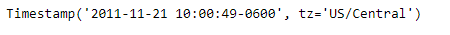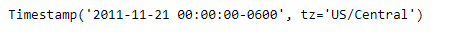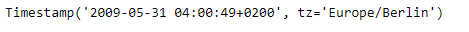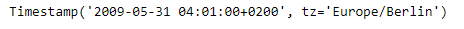# Python | Pandas Timestamp.round

• Last Updated : 14 Jan, 2019

Python is a great language for doing data analysis, primarily because of the fantastic ecosystem of data-centric python packages. Pandas is one of those packages and makes importing and analyzing data much easier.

Pandas` Timestamp.round()` function is used to round the given Timestamp to the specified resolution.

Syntax :Timestamp.round()

Parameters :
freq : a freq string indicating the rounding resolution

Return : a new Timestamp rounded to the given resolution of freq

Example #1: Use `Timestamp.round()` function to round the given Timestamp to Daily time series frequency.

 `# importing pandas as pd``import` `pandas as pd`` ` `# Create the Timestamp object``ts ``=` `pd.Timestamp(year ``=` `2011``,  month ``=` `11``, day ``=` `21``,``                  ``hour ``=` `10``, second ``=` `49``, tz ``=` `'US/Central'``)`` ` `# Print the Timestamp object``print``(ts)`

Output :Now we will use the `Timestamp.round()` function to round the given Timestamp object to Daily frequency.

 `# round to daily frequency``ts.``round``(freq ``=` `'D'``)`

Output :As we can see in the output, the `Timestamp.round()` function has returned a Timestamp object having the time series frequency rounded to the desired resolution.

Example #2: Use `Timestamp.round()` function to round the given Timestamp to minutely time series frequency.

 `# importing pandas as pd``import` `pandas as pd`` ` `# Create the Timestamp object``ts ``=` `pd.Timestamp(year ``=` `2009``, month ``=` `5``, day ``=` `31``,``       ``hour ``=` `4``, second ``=` `49``, tz ``=` `'Europe/Berlin'``)`` ` `# Print the Timestamp object``print``(ts)`

Output :Now we will use the `Timestamp.round()` function to round the given Timestamp object to minutely frequency.

 `# round to minutely frequency``ts.``round``(freq ``=` `'T'``)`

Output :As we can see in the output, the `Timestamp.round()` function has returned a Timestamp object having the time series frequency rounded to the desired resolution.

My Personal Notes arrow_drop_up